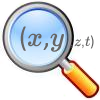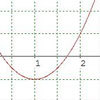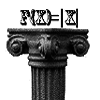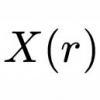# Resources tagged with: Calculus generally

Filter by: Content type:
Age range:
Challenge level:

There are 27 NRICH Mathematical resources connected to Calculus generally, you may find related items under Calculus.

Broad Topics > Calculus > Calculus generally### Interactive Workout - Mathmo

##### Age 16 to 18 ShortChallenge Level

Mathmo is a revision tool for post-16 mathematics. It's great installed as a smartphone app, but it works well in pads and desktops and notebooks too. Give yourself a mathematical workout!### Population Dynamics Collection

##### Age 16 to 18Challenge Level

This is our collection of tasks on the mathematical theme of 'Population Dynamics' for advanced students and those interested in mathematical modelling.### Population Dynamics - Part 1

##### Age 16 to 18Challenge Level

First in our series of problems on population dynamics for advanced students.### A Different Differential Equation

##### Age 16 to 18Challenge Level

Explore the properties of this different sort of differential equation.### Population Ecology Using Probability

##### Age 16 to 18Challenge Level

An advanced mathematical exploration supporting our series of articles on population dynamics for advanced students.### Population Dynamics - Part 5

##### Age 16 to 18Challenge Level

Fifth in our series of problems on population dynamics for advanced students.### Population Dynamics - Part 6

##### Age 16 to 18Challenge Level

Sixth in our series of problems on population dynamics for advanced students.### Branching Processes and Extinction

##### Age 16 to 18Challenge Level

An advanced mathematical exploration supporting our series of articles on population dynamics for advanced students.### Population Dynamics - Part 4

##### Age 16 to 18Challenge Level

Fourth in our series of problems on population dynamics for advanced students.### Patterns of Inflection

##### Age 16 to 18Challenge Level

Find the relationship between the locations of points of inflection, maxima and minima of functions.### Population Dynamics - Part 2

##### Age 16 to 18Challenge Level

Second in our series of problems on population dynamics for advanced students.### Calculus Analogies

##### Age 16 to 18Challenge Level

Consider these analogies for helping to understand key concepts in calculus.### Curve Hunter

##### Age 14 to 18Challenge Level

This problem challenges you to sketch curves with different properties.### Turning to Calculus

##### Age 16 to 18Challenge Level

Get started with calculus by exploring the connections between the sign of a curve and the sign of its gradient.### Population Dynamics - Part 3

##### Age 16 to 18Challenge Level

Third in our series of problems on population dynamics for advanced students.### Maths Shop Window

##### Age 16 to 18Challenge Level

Make a functional window display which will both satisfy the manager and make sense to the shoppers### Erratic Quadratic

##### Age 16 to 18Challenge Level

Can you find a quadratic equation which passes close to these points?### Scientific Curves

##### Age 16 to 18Challenge Level

Can you sketch these difficult curves, which have uses in mathematical modelling?### Calculus Countdown

##### Age 16 to 18Challenge Level

Can you hit the target functions using a set of input functions and a little calculus and algebra?### Operating Machines

##### Age 16 to 18Challenge Level

What functions can you make using the function machines RECIPROCAL and PRODUCT and the operator machines DIFF and INT?### Implicitly

##### Age 16 to 18Challenge Level

Can you find the maximum value of the curve defined by this expression?### Integral Arranging

##### Age 16 to 18Challenge Level

How would you sort out these integrals?### Fractional Calculus III

##### Age 16 to 18

Fractional calculus is a generalisation of ordinary calculus where you can differentiate n times when n is not a whole number.### Fractional Calculus II

##### Age 16 to 18

Here explore some ideas of how the definitions and methods of calculus change if you integrate or differentiate n times when n is not a whole number.### Fractional Calculus I

##### Age 16 to 18

You can differentiate and integrate n times but what if n is not a whole number? This generalisation of calculus was introduced and discussed on askNRICH by some school students.### Power Up

##### Age 16 to 18Challenge Level

Show without recourse to any calculating aid that 7^{1/2} + 7^{1/3} + 7^{1/4} < 7 and 4^{1/2} + 4^{1/3} + 4^{1/4} > 4 . Sketch the graph of f(x) = x^{1/2} + x^{1/3} + x^{1/4} -x### 2D-3D

##### Age 16 to 18Challenge Level

Two circles of equal size intersect and the centre of each circle is on the circumference of the other. What is the area of the intersection? Now imagine that the diagram represents two spheres of. . . .